# 5.6: Phase Shift of Sinusoidal Functions

•• Contributed by CK12
• CK12

A periodic function that does not start at the sinusoidal axis or at a maximum or a minimum has been shifted horizontally. This horizontal movement allows for different starting points since a sine wave does not have a beginning or an end.

What are five other ways of writing the function $$f(x)=2 \cdot \sin x ?$$

### Phase Shift of Sinusoidal Functions

The general sinusoidal function is:

$$f(x)=\pm a \cdot \sin (b(x+c))+d$$

The constant $$c$$ controls the phase shift. Phase shift is the horizontal shift left or right for periodic functions. If $$c=\frac{\pi}{2}$$ then the sine wave is shifted left by $$\frac{\pi}{2}$$. If $$c=-3$$ then the sine wave is shifted right by $$3 .$$ This is the opposite direction than you might expect, but it is consistent with the rules of transformations for all functions.

To graph a function such as $$f(x)=3 \cdot \cos \left(x-\frac{\pi}{2}\right)+1,$$ first find the start and end of one period. Then sketch only that portion of the sinusoidal axis. Finally, plot the 5 important points for a cosine graph while keeping the amplitude in mind. The graph is shown below.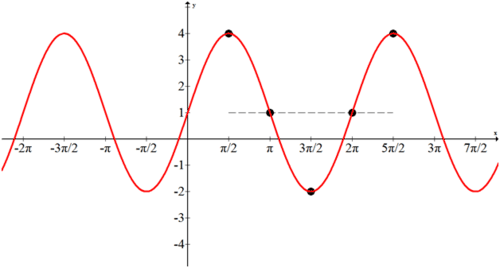Generally $$b$$ is always written to be positive. If you run into a situation where $$b$$ is negative, use your knowledge of even and odd functions to rewrite the function.

$$\cos (-x)=\cos (x)$$
$$\sin (-x)=-\sin (x)$$

### Examples

Example 1

Earlier, you were asked to write $$f(x)=2 \cdot \sin x$$ in five different ways. The function $$f(x)=2 \cdot \sin x$$ can be rewritten an infinite number of ways.

$$2 \cdot \sin x=-2 \cdot \cos \left(x+\frac{\pi}{2}\right)=2 \cdot \cos \left(x-\frac{\pi}{2}\right)=-2 \cdot \sin (x-\pi)=2 \cdot \sin (x-8 \pi)$$

It all depends on where you choose start and whether you see a positive or negative sine or cosine graph.

Example 2

Given the following graph, identify equivalent sine and cosine algebraic models.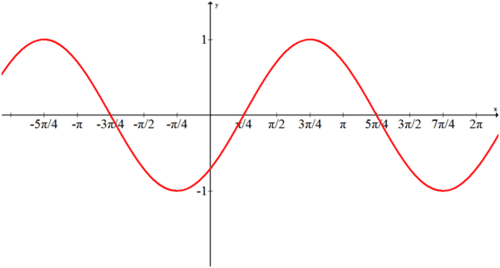Either this is a sine function shifted right by $$\frac{\pi}{4}$$ or a cosine graph shifted left $$\frac{5 \pi}{4}$$.

$$f(x)=\sin \left(x-\frac{\pi}{4}\right)=\cos \left(x+\frac{5 \pi}{4}\right)$$

Example 3

At $$t=5$$ minutes William steps up 2 feet to sit at the lowest point of the Ferris wheel that has a diameter of 80 feet. A full hour later he finally is let off the wheel after making only a single revolution. During that hour he wondered how to model his height over time in a graph and equation.

Since the period is 60 which works extremely well with the $$360^{\circ}$$ in a circle, this problem will be shown in degrees.

$$\begin{array}{|l|l|} \hline \text { Time (minutes) } & \text { Height (feet) } \\ \hline 5 & 2 \\ \hline 20 & 42 \\ \hline 35 & 82 \\ \hline 50 & 42 \\ \hline 65 & 2 \\ \hline \end{array}$$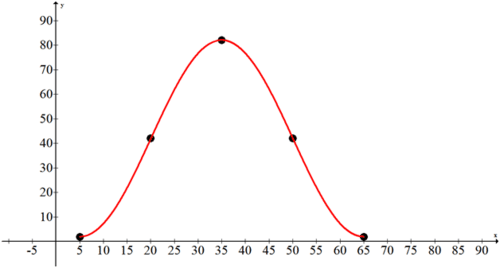William chooses to see a negative cosine in the graph.  He identifies the amplitude to be 40 feet.  The vertical shift of the sinusoidal axis is 42 feet.  The horizontal shift is 5 minutes to the right

The period is 60 (not 65 ) minutes which implies $$b=6$$ when graphed in degrees.

$$60=\frac{360}{b}$$

Thus one equation would be:

$$f(x)=-40 \cdot \cos (6(x-5))+42$$

Example 4

Tide tables report the times and depths of low and high tides.  Here is part of tide report from Salem, Massachusetts dated September 19, 2006.

$$\begin{array}{|c|c|c|} \hline 10: 15 \mathrm{AM} & 9 \mathrm{ft} & \text { High Tide } \\ \hline 4: 15 \mathrm{PM} & 1 \mathrm{ft} . & \text { Low Tide } \\ \hline 10: 15 \mathrm{PM} & 9 \mathrm{ft} & \text { High Tide } \\ \hline \end{array}$$

Find an equation that predicts the height based on the time. Choose when $$t=0$$ carefully.

There are two logical places to set $$t=0$$. The first is at midnight the night before and the second is at 10: 15 AM. The first option illustrates a phase shift that is the focus of this concept, but the second option produces a simpler equation. Set $$t=0$$ to be at midnight and choose units to be in minutes.

$$\begin{array}{|l|l|l|} \hline \text { Time (hours : minutes) } & \text { Time (minutes) } & \text { Tide (feet) } \\ \hline 10: 15 & 615 & 9 \\ \hline 16: 15 & 975 & 1 \\ \hline 22: 15 & 1335 & 9 \\ \hline & \frac{615+975}{2}=795 & 5 \\ \hline & \frac{1335+975}{2}=1155 & 5 \\ \hline \end{array}$$

These numbers seem to indicate a positive cosine curve.  The amplitude is 4 and the vertical shift is 5.  The horizontal shift is 615 and the period is 720.

$$720=\frac{2 \pi}{b} \rightarrow b=\frac{\pi}{360}$$

Thus one equation is:

$$f(x)=4 \cdot \cos \left(\frac{\pi}{360}(x-615)\right)+5$$

Example 5

Use the equation from Example 4 to find out when the tide will be at exactly $$8 \mathrm{ft}$$ on September $$19^{t h}$$.

This problem gives you the $$y$$ and asks you to find the $$x$$. Later you will learn how to solve this algebraically, but for now use the power of the intersect button on your calculator to intersect the function with the line $$y=8$$. Remember to find all the $$x$$ values between 0 and 1440 to account for the entire 24 hours.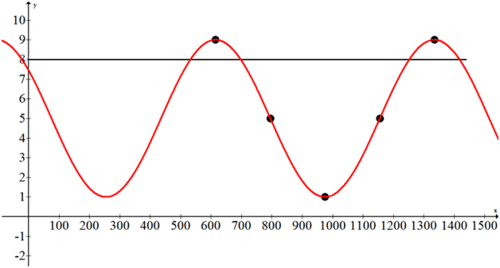There are four times within the 24 hours when the height is exactly 8 feet.  You can convert these times to hours and minutes if you prefer.

$$t \approx 532.18$$ (8:52), 697.82 (11:34), 1252.18 (20:52), 1417.82 (23:38)

Review

Graph each of the following functions.

1. $$f(x)=2 \cos \left(x-\frac{\pi}{2}\right)-1$$

2. $$g(x)=-\sin (x-\pi)+3$$

3. $$h(x)=3 \cos (2(x-\pi))$$

4. $$k(x)=-2 \sin (2 x-\pi)+1$$

5. $$j(x)=-\cos \left(x+\frac{\pi}{2}\right)$$

Give one possible sine equation for each of the graphs below.

6.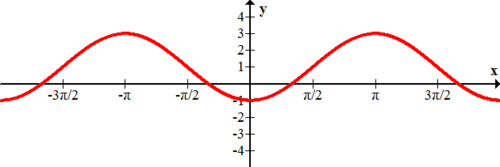7.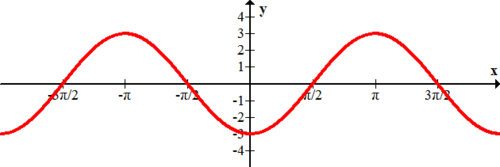8.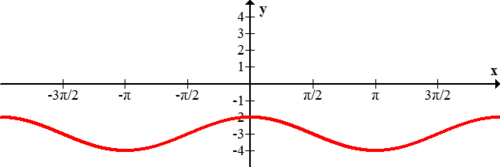Give one possible cosine function for each of the graphs below.

9.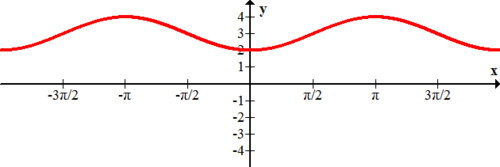10.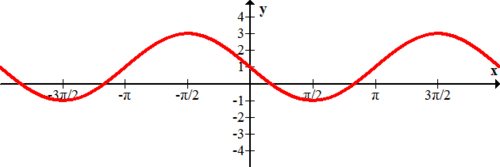11.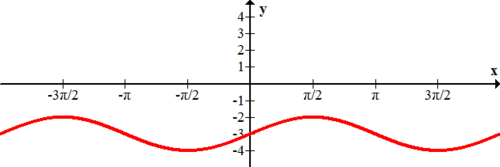The temperature over a certain 24 hour period can be modeled with a sinusoidal function. At 3: 00 , the temperature for the period reaches a low of $$22^{\circ} \mathrm{F}$$. At $$15: \mathrm{OO}$$, the temperature for the period reaches a high of $$40^{\circ} F$$

12. Find an equation that predicts the temperature based on the time in minutes. Choose $$t=0$$ to be midnight.

13. Use the equation from #12 to predict the temperature at $$4: 00 \mathrm{PM}$$.

14. Use the equation from #12 to predict the temperature at 8: 00 AM.

15. Use the equation from #12 to predict the time(s) it will be $$32^{\circ} \mathrm{F}$$.

...